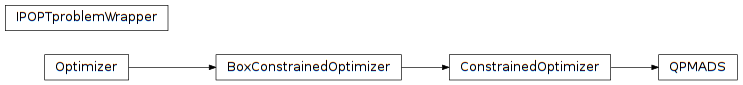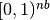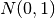# 4.12. pyopus.optimizer.qpmads — Mesh adaptive direct search with quadratic programming¶Mesh Adaptive Direct Search with quadratic programming (PyOPUS subsystem name: MADSOPT)

Mesh adaptive direct search based on [mads]. The 2n version uses orthogonal directions [madsort]. Handles infeasible initial points with the progressive barrier approach [madspb].

Supports uniform distribution of the search directions according to [unimads].

Builds and solves quadratic models based on [qpmads].

 [mads] Audet C., Dennis Jr. J.E., Mesh Adaptive Direct Search Algorithms for Constrained Optimization. SIAM Journal on Optimization, vol. 17, pp. 188-217, 2006.
 [madsort] Abramson M.A., Audet C., Dennis Jr. J.E., Le Digabel S., ORTHO-MADS: A Deterministic MADS Instance with Orthogonal Directions. SIAM Journal on Optimization, vol. 20, pp.948-966,2009.
 [madspb] Audet C., Dennis Jr. J.E., A Progressive Barrier for Derivative-Free Nonlinear Programming. SIAM Journal on Optimization, vol. 20, pp. 445-472, 2009.
 [unimads] Bűrmen Á., Tuma T., Generating Poll Directions for Mesh Adaptive Direct Search with Realizations of a Uniformly Distributed Random Orthogonal Matrix, Pacific Journal of Optimization, acepted for publication, 2015.
 [qpmads] Bűrmen Á., Olenšek J., Tuma T., Mesh Adaptive Direct Search with Second Directional Derivative-Based Hessian Update, Computational Optimization and Applications, DOI: 10.1007/s10589-015-9753-5, 2015.

Not all member functions are documented because this module is under development.

class pyopus.optimizer.qpmads.QPMADS(function, xlo=None, xhi=None, constraints=None, clo=None, chi=None, fc=None, cache=False, fgH=None, cJ=None, debug=0, fstop=None, maxiter=None, scaling=1.0, meshBase=4.0, initialMeshDensity=1.0, stepBase=2.0, startStep=1.0, maxStep=inf, stopStep=1e-12, generator=2, qraugment=0, unifmat=0, protoset=1, rounding=1, sequenceReset=False, boundSnap=False, boundSlide=False, roundOnFinestMeshEntry=False, greedy=True, speculativePollSearch=True, fabstol=0.0, freltol=1.1102230246251565e-16, xabstol=0.0, xreltol=1.1102230246251565e-16, Habstol=None, Hreltol=None, HinitialDiag=0.0, model=True, linearUpdate=True, simplicalUpdate=False, powellUpdate=False, forceRegression=True, boundStepMirroring=True, linearUpdateExtend=True, rho=4.0, lastDirectionOrdering=False, modelOrdering=True, modelSearch=True, beta=0.001, rhoNeg=inf, qpFeasibilityRestoration=False, speculativeModelSearch=False, stepCutAffectsUpdate=True, speculativeStepAffectsUpdate=False, hmax=0.0)

Mesh Adaptive Direct Search

See the ConstrainedOptimizer for the explanation of function, xlo, xhi, constraints, clo, chi, fc, cache, debug, fstop, and maxiter.

fgH specifies a function that evaluates the function gradient and Hessian. The function should return them as a tuple with gradient as the first component and the Hessian as the second component.

cJ specifies a function that computes the Jacobian of the nonlinear constraints. The function returns a Numpy array with first index for the constraint and the second index for the optimization variable.

scaling is the scaling of the problem. If it is scalar, it applies to all components of the independent variable. If it is a vector scaling can be specified independently for every component.

meshBase is the base for constructing the mesh. Mesh densities are powers of thin number.

initialMeshDensity specifies the density of the initial mesh. The mesh size is obtained by dividing the power of the meshBase with this number.

stepbase is the base for constructing the step size. Steps size is computed as some constant times a power of this number.

startStep is the initial step size. The exponents of the initial mesh and step size is chosen in such manner that the initial step closely approximates this number.

maxStep is the upper limit for step size.

stopStep is the step size at which the algorithm stops.

generator is the direction generator method.

• 0 – OrthoMADS
• 1 – QRMADS
• 2 – UniMADS (uniformly distributed directions)

unifmat is the matrix construction method for QRMADS and UniMADS. This matrix is used as the input of the QR decomposition. nb is the smallest even number not greater than n.

• 0 – n independent random samples from• 1 – n samples from nb-dimensional Sobol sequence, advance generator by one sample
• 2 – 1 sample from nb-dimensional Sobol sequence and n random samples from• 3 – n+1 samples from nb-dimensional Sobol sequence, advance generator by one sample
• 4 – n+1 independent random samples from• 5 – one sample from nb(n+1)-dimensional Sobol sequence (take nb rows, n columns)
• 6 – one sample from nb(n+1)-dimensional Sobol sequence (take nb rows, n+1 columns)

qraugment is the matrix augmentation method for QRMADS. The first column is always transformed with the Box-Muller transformation resulting in a vector that (when normalized) is uniformly distributed on the unit sphere. The remaining columns are transformed in the following manner:

• 0 – matrix elements transformed to uniform distribution over [-1,1]
• 1 – matrix elements transformed to uniform distribution over [0,1]
• 2 – matrix elements transformed to normal distribution• 3 – same as 2, except that the first column is fixed (the first element is 1, the remaining elements are 0)

qraugment does not affect UniMADS. For UniMADS all matrix elements are transformed to normal distributionbefore QR decomposition takes place.

protoset specifies the prototype set for UniMADS

• 0 – regular simplex with n+1 points
• 1 – n orthogonal vectors and their negatives

rounding specifies the rounding mode for QRMADS and UniMADS.

• 0 – round to sphere
• 1 – round to hypercube

Two generators are created for generating random/Halton/Sobol sequences. The main generator is used for most iterations, while the auxiliary generator is used for the iterations involving the finest-yet meshes. If sequencereset is True the main generator is reset to the state of the auxiliary generator whenever the finest-yet mesh is reached.

Setting boundSlide to True sets the components of a point that violate bound to the value of the respective bound. This makes the point feasible.

Setting boundSnap to True shortens the all steps that result in bound violation to such extent that the resulting point lies on the bound with largest violation. This makes the point feasible.

Setting roundOnFinestMeshEntry to True rounds the points examined on the finest-yet mesh to the nearest mesh point. Otherwise these points are not rounded.

greedy turns on greedy evaluation in the poll step (stops the poll step as soon as a better point is found).

speculativePollSearch enables the speculative step after a successful poll step.

fabstol and freltol specify the absolute and the relative error of the computed function value used for estimating the error of the Hessian update.

xabstol and xreltol specify the absolute and the relative error of the point position used for estimating the error of the Hessian update.

Habstol and Hreltol specify the maximal absolute and relative error of the Hessian update for which the update is applied. Setting them to None applies the update regardless of the error.

HinitialDiag specifies the diagonal elements of the initial hessian approximation. If this is a scalar all diagonal elements of the initial approximation are identical.

Setting model to True turns on the construction of the quadratic model of the function and constraints.

Setting linearUpdate to True turns on Hessian updating based on three collinear points. This type of updating works well with protoset set to 1.

Setting simplicalUpdate to True turns on Hessian updating based on n+2 points. Works well for protoset set to 0.

powellUpdate turns on experimental Hessian updating based on minimum Frobenius norm updating developed by Powell.

forceRegression forces the computation of the gradients based on linear regression for the powellUpdate. For other updates the gradients are computed using linear regression.

boundStepMirroring turns on mirroring of points that violate bounds.

linearUpdateExtend evaluates an additional expansion point if a point violates the bound. useful with protoset set to 1. If direction d violates bounds and -d does not an additional point along direction -2d is evaluated. This results in 3 collinear points that make it possible to apply the linearUpdate.

rho specifies the distance within which points are collected for regression.

lastDirectionOrdering enables the ordering of poll steps based on the last successful direction.

modelOrdering enables the ordering of poll steps based on the quadratic model.

modelSearch turns on quadratic model-based search (uses CVXOPT). This search is also referred to as the QP search.

beta is the parameter used in the algorithm for finding a positive definite Hessian.

rhoNeg is the trust region radius used when the Hessian is not positive definite.

Setting qpFeasibilityRestoration to True shrinks the QP computed step so that it satisfies the model constraints.

speculativeModelSearch turns on the speculative step after a successful QP serach step.

Setting hmax to a nonzero value turns on filter-based point acceptance which makes it possible to swart from an infeasible point that violates the nonlinear constraints.

If stepCutAffectsUpdate is enabled a step cut caused by bound sliding or bound snapping prevents the mesh and the step size from increasing, even when the step is successful.

If speculativeStepAffectsUpdate is enabled a rejected speculative step prevents the mesh and the step size from increasing.

See the Optimizer class for more information.

boxMuller(v)

Box-Muller transformation of a vector / matrix columns.

check()

Checks the optimization algorithm’s settings and raises an exception if something is wrong.

collect_powell()

Used together with the Powell update.

Copies the gradient, the Hessian, and the Jabobian from the internal structures of the update procedure.

Returns the gradient of objective and the Jacobian of the constraints.

explicit_gHJ(x0)

Computes the explicitly given gradients and Hessian.

generatePollSteps(reduction=False)

Generates a scaled and rounded set of poll steps.

method can be
0 – original OrthoMADS 1 – QRMADS 2 – UniMADS 3 – 2 opposite vectors
gridRestrain(x, avoidOrigin=False)

Returns a point restrained to the current grid. If avoidOrigin is True rounds away from the origin.

The point is a normalized point (i.e. needs to be multiples by self.scaling to get the actual step).

modelOrderSteps(x0, p, H, g, J=None, c=None, reverse=False)

Order steps p according to quadratic model given by H and g.

If J and c are given linearized constraints of the form clo<=Jx+c<=chi are considered as the primary criterion for ordering.

If reverse is True considers pairs of the forms (p, -p) first. After that the resulnting points are ordered.

Note that steps are normalized steps. The model applies to normalized steps. Actual steps are obtained by scaling p with self.scaling.

orderSteps(p, refDir, reverse=False)

Orders the steps in ascending angle order with respect to refDir.

If reverse is True, steps are reversed when angle is greater than pi/2.

Note that steps are normalized steps. Actual steps are obtained by multiplying them with self.scaling.

reset(x0)

Puts the optimizer in its initial state and sets the initial point to the 1-dimensional array x0. The length of the array becomes the dimension of the optimization problem (ndim member). The length of x must match that of xlo and xhi.

restoreFeasibility(x0, d, H=None, g=None, J=None, c=None, boundCheck=True, boundSlide=False, rounding=True)
Restore feasibility by shrinking d until x0 + d * self.scaling
satisfies bounds reduces the model (if H and g are given) satisfies linearized constraints (if J and c are given)

Note that the model applies to the normalized step.

Applies rounding to d if rounding is set to True.

Returns final scaled point, the used shrink factor a, and a flag indicating sliding was used.

run()

Runs the optimization algorithm.

#### Previous topic

4.11. pyopus.optimizer.psade — Box constrained parallel SADE global optimizer

#### Next topic

5. pyopus.problems — Test problems for optimizaion algorithms